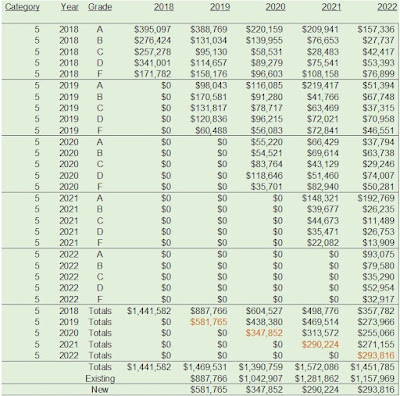## January 02, 2022

Let's assume you retain 33% of last year's customer base. If you retain the customer, the customer spends \$200. Let's assume you have 100,000 twelve-month buyers.

Let's assume that each new customer spends \$120 in the year of acquisition. Let's assume you generate 70,000 new/reactivated buyers a year.

Let's assume your CEO somehow commands you to increase retention rates by 20% ... meaning that your 33% rebuy rate becomes a 39.6% rebuy rate.

• 12-Month Buyers = 39.6% Rebuy Rate * \$200 per Repurchaser * 100,000 Customers = \$7,920,000.
• New/Reactivated Buyers = 70,000 * \$120 = \$8,400,000.
• Total Sales = \$7,920,000 + \$8,400,000 = \$16,320,000.
• Total Customers = 39,600 + 70,000 = 109,600.
Now, let's assume your CEO instead commands you to increase new/reactivated customer counts by 20%.

• 12-Month Buyers = 33% Rebuy Rate * \$200 per Repurchaser * 100,000 Customers = \$6,600,000.
• New/Reactivated Buyers = 70,000*1.2 * \$120 = \$10,080,000.
• Total Sales = \$7,920,000 + \$8,400,000 = \$16,680,000.
• Total Customers = 33,000 + 84,000 = 117,000.
• \$16,320,000 annual sales and 109,600 twelve-month buyers?
• \$16,680,000 annual sales and 117,000 twelve-month buyers?
We'd all pick the latter, every one of us.

And yet, we constantly chase the former.

Our focus today determines how successful we'll be tomorrow. Let's focus on what matters.